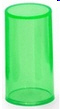KG2
 Name:    KG2

Multiple Choice
Identify the choice that best completes the statement or answers the question.

1.

Which shape is a triangle?
 a.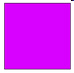c.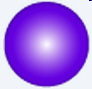b.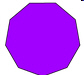d.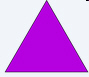2.

Which shape is a cube?
 a.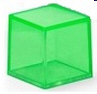c.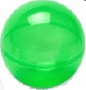b.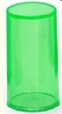d.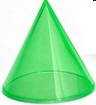3.

Which shape is a cone?
 a.c.b.d.4.

Which shape is a sphere?
 a.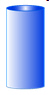c.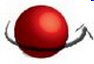b.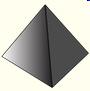d.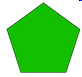5.

Which shape is a rectangle?
 a.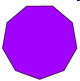c.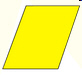b.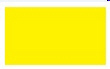d.6.

Which shape is an oval?
 a.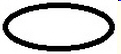c.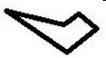b.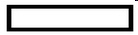d.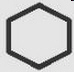7.

Which shape is a square?
 a.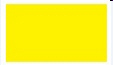c.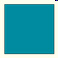b.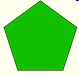d.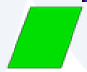8.

Which shape is a star?
 a.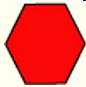c.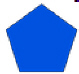b.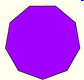d.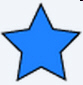9.

Which shape is a circle?
 a.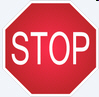c.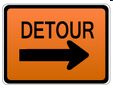b.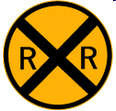d.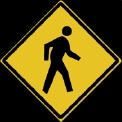10.

Which shape is a cylinder?
 a.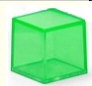c.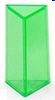b.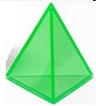d.